# Fraction minimal working exemple

This file is a report about a small bug in geometry.h in the function line_length_center. If the Level-Set function used to define the volume fraction nullifies exactly between two cells, the length of the interface is set to 0 in both cells, whereas it should return 1 in one of the cells. This seems due to a marginal case, not treated in the function line_length_center.

If the interface is given by Phi = y, the normal vector n is (0, 1) and alpha = +/- 0.5 in bottom (resp top) cells.

At the line 354-361 of geometry.h, alpha is translated with the normal vector. In our case, we obtain alpha = 0 in one cell (top) and alpha = 1 in the other cell (bottom). The case is eliminated with every cells without interface at the line 365 and 0 is returned.

#include "grid/multigrid.h"
#include "fractions.h"

We set LEVEL to 2 to have a small number of cells and better see the bug.

const int LEVEL = 2;

int main() {

The orgin is set at the center of the domain.

  origin (- 0.5, - 0.5);
N = 1 << LEVEL;
init_grid (N);

Two fractions are defined and computed successively :

• First corresponding to the Level Set function y;
• Second corresponding to the Level Set function y+1e-3;
  scalar f[], L[], alpha[], nT[];
for (int i = 0; i <= 1; i++) {
fraction (f, y + i*1e-3);
boundary ({f});

The length of the interface is computed in each cell.

    foreach() {
coord coord_centroid = {0, 0};
coord n = mycs (point, f);
alpha[] = line_alpha (f[], n);
nT[] = n.x ;
L[] = Delta * line_length_center(n, alpha[], &coord_centroid);
}

The cell grid, values of the fractions and interfacial length are saved.

#if i == 0
FILE * fp = fopen ("cells", "w");
output_cells (fp);
fclose (fp);
#endif

fp = fopen (i ? "facets_1" : "facets_0", "w");
output_facets(f, fp);
fclose (fp);

fp = fopen (i ? "fvalues_1" : "fvalues_0", "w");
foreach()
fprintf(fp,"%g %g %g %g %g %g\n", x, y, f[], alpha[], nT[], L[]);
fclose (fp);
}
}

## Ouputs

 set output 'plot1.png'
unset key
unset border
unset tics
plot \
'cells' w l lc rgb "#7F7F7F", \
'facets_0' u 1:2 w filledcu, \
'fvalues_0' u 1:2:3 with labels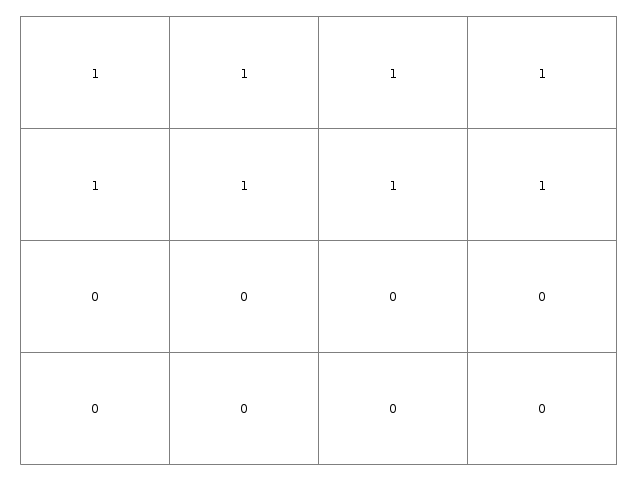Reconstruction of \phi(x, y) = y (script)

 set output 'plot2.png'
plot \
'cells' w l lc rgb "#7F7F7F", \
'facets_1' u 1:2 w l, \
'fvalues_1' u 1:2:3 with labels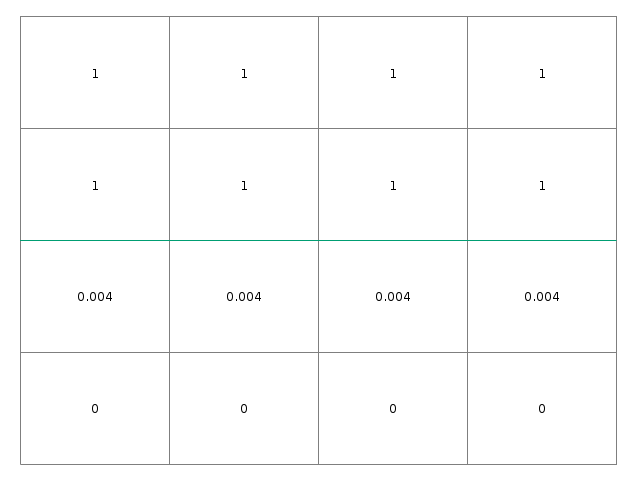Reconstruction of \phi(x, y) = y + 1e-3 (script)

The volume fraction is correct in both cases.

 set output 'plot3.png'
plot \
'cells' w l lc rgb "#7F7F7F", \
'facets_0' u 1:2 w l, \
'fvalues_0' u 1:2:4 with labels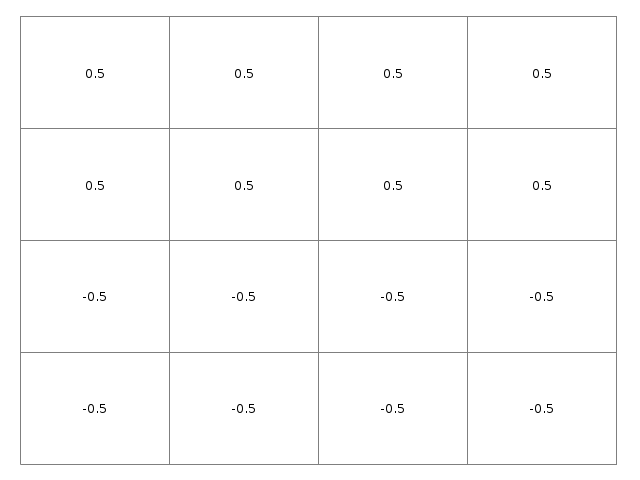Alpha value for \phi(x, y) = y (script)

 set output 'plot4.png'
plot \
'cells' w l lc rgb "#7F7F7F", \
'facets_1' u 1:2 w l, \
'fvalues_1' u 1:2:4 with labels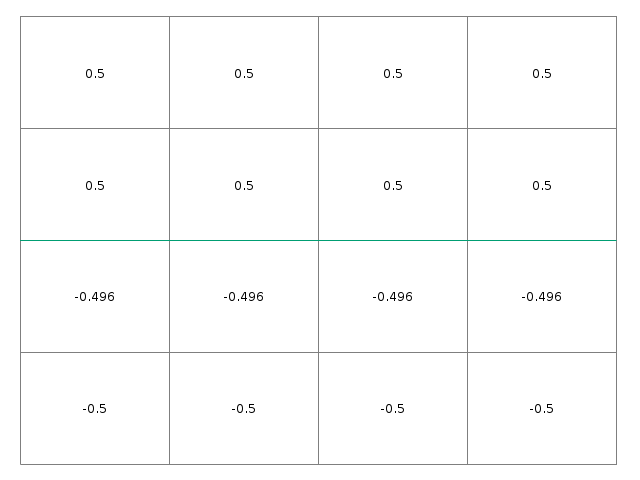Alpha value for \phi(x, y) = y + 1e-3 (script)

The alpha values are correctly computed as well in both cases

 set output 'plot5.png'
plot \
'cells' w l lc rgb "#7F7F7F", \
'facets_0' u 1:2 w l, \
'fvalues_0' u 1:2:6 with labels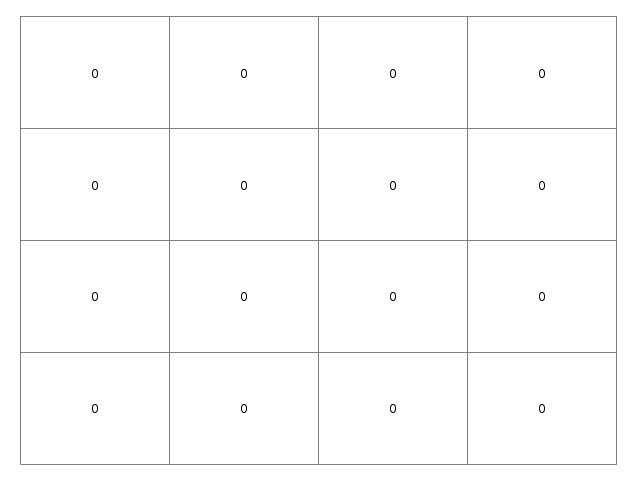Interfacial length for \phi(x, y) = y (script)

 set output 'plot6.png'
plot \
'cells' w l lc rgb "#7F7F7F", \
'facets_1' u 1:2 w l, \
'fvalues_1' u 1:2:6 with labels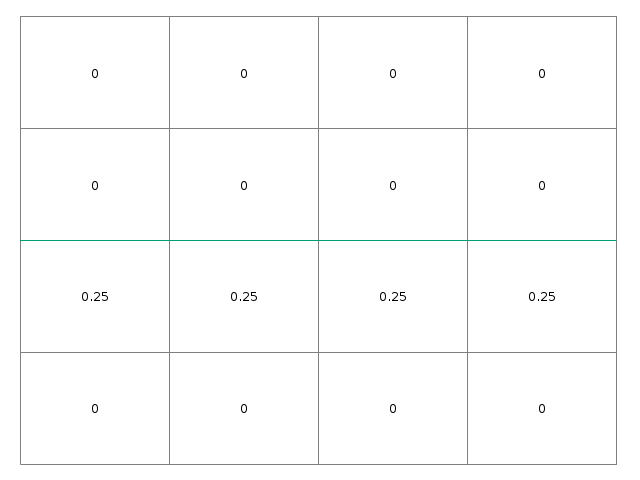Interfacial length for \phi(x, y) = y + 1e-3 (script)

The interfacial lengths are not correct in the case y = 0.